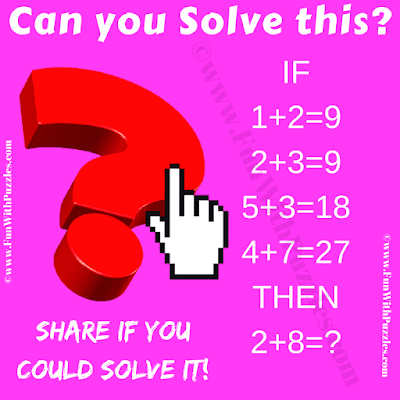This is a very interesting and brain-twisting mathematical reasoning question.  In this brain teaser, you are shown some logical reasoning equations. Your challenge is to crack the logical reasoning hidden in these number equations. Once you are able to find the hidden logic in these equations, then find the value of the missing number which will replace the question mark. Let's see if you can crack this logical code?Can you find the value of the missing number?
The answer to this "Mathematical Reasoning Question Brain Teaser", can be viewed by clicking on the answer button.